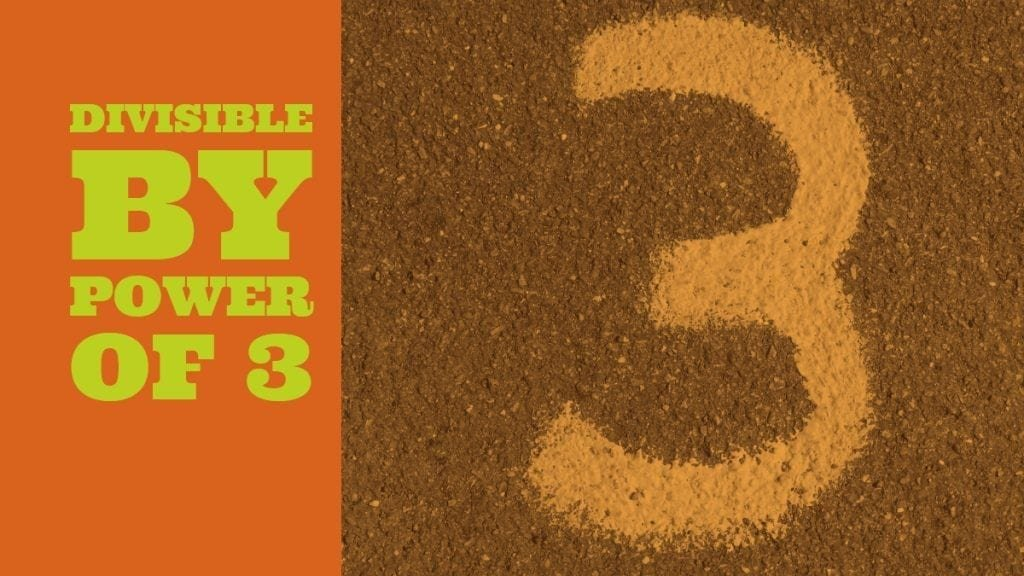How Cheenta works to ensure student success?
Explore the Back-Story

# Test of Mathematics Solution Subjective 32 | Power of 3Test of Mathematics Solution Subjective 32 (from ISI Entrance). The book, Test of Mathematics at 10+2 Level is Published by East West Press. This problem book is indispensable for the preparation of I.S.I. B.Stat and B.Math Entrance.

## Problem

Show that the number 11...1 with $3^n$ digits is divisible by $3^n$

## Solution

We use induction. For n=1; we check 111 is divisible by 3. Assuming that the result host for n=k, we establish that it holds for n=k+1.

The number 111...111 (with $3^{k+1}$ digits) can be written in 3 blocks each having $3^{k}$ 1's.  Hence we can write it as ${111...111} \times 10^{(2 \times 3^k)} + {111...111} \times 10^{(3^k)} + {111...111}$ where {111...111} denotes $3^k$ 1's.
Taking {111...111} common we have $(111...111)(10^{(2 \cdot 3^k)} + 10^{(3^k)} + 1)$. By induction (111...111) is divisible by $3^k$ and we also have 3 dividing $(10^{(2 \cdot 3^k)} + 10^{(3^k)} +1 )$ as it's sum of digits is 3 (it has only three 1's and rest are 0) .
Hence (111...111) having $3^{(k+1)}$ 1's is divisible by $3^{(k+1)}$.Test of Mathematics Solution Subjective 32 (from ISI Entrance). The book, Test of Mathematics at 10+2 Level is Published by East West Press. This problem book is indispensable for the preparation of I.S.I. B.Stat and B.Math Entrance.

## Problem

Show that the number 11...1 with $3^n$ digits is divisible by $3^n$

## Solution

We use induction. For n=1; we check 111 is divisible by 3. Assuming that the result host for n=k, we establish that it holds for n=k+1.

The number 111...111 (with $3^{k+1}$ digits) can be written in 3 blocks each having $3^{k}$ 1's.  Hence we can write it as ${111...111} \times 10^{(2 \times 3^k)} + {111...111} \times 10^{(3^k)} + {111...111}$ where {111...111} denotes $3^k$ 1's.
Taking {111...111} common we have $(111...111)(10^{(2 \cdot 3^k)} + 10^{(3^k)} + 1)$. By induction (111...111) is divisible by $3^k$ and we also have 3 dividing $(10^{(2 \cdot 3^k)} + 10^{(3^k)} +1 )$ as it's sum of digits is 3 (it has only three 1's and rest are 0) .
Hence (111...111) having $3^{(k+1)}$ 1's is divisible by $3^{(k+1)}$.

### Knowledge Partner